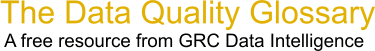Choose Index below for a list of all words and phrases defined in this glossary.

# Co-ordinate/Coordinate System

index | Index

## Co-ordinate/Coordinate system - definitions

coordinate system - Composed of a set of coordinate axes with a known metric. The concept 'metric of a coordinate space' consists of the set of mathematical rules that defines the relationships between the coordinate values and the invariant spatial quantities between points; for example, the mathematical rules (formulae) required for calculating angles and distances between points from coordinate values and vice versa.

[Category=Geospatial ]

Source: Open Geospatial Consortium, 06 June 2011 07:28:51, http://www.opengeospatial.org/ogc/glossaryThese advertisers support this free service

coordinate system - [coordinate systems] A reference framework consisting of a set of points, lines, and/or surfaces, and a set of rules, used to define the positions of points in space in either two or three dimensions. The Cartesian coordinate system and the geographic coordinate system used on the earth's surface are common examples of coordinate systems.

[Category=Geospatial ]

Source: esri, 08 March 2012 08:35:46, http://support.esri.com/en/knowledgebase/GISDictionary/term/abbreviationData Quality Glossary.  A free resource from GRC Data Intelligence. For comments, questions or feedback: dqglossary@grcdi.nl